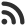Feed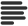Articles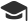Tutorials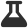Lab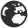CompaniesLeaderboard
 DG Student at BIT Mesra Aug. 21, 2020, 11:41 a.m. ⋅ 2416 views

# Deloitte Placement paper Aptitude Questions with answers

1. Length of the rectangular field is 4 times the breadth. The area of rectangular field is 2500 Yards. Find the length of the field.

A. 100

B. 25

C. 50

D. 150

Ans. A

2. Find the probability of getting at least one tail if two coins are tossed simultaneously.

A. 3/2

B. 3/8

C. 15/8

D. 3/4

Ans. D

3. There are 4 raspberries and 8 strawberries. Find the probability if two fruits are chosen and both are raspberries.

A. 1/2

B. 1/11

C. 1/6

D. 2/7

Ans. C

4. If b is the simple interest on a and c is the simple interest on b at same period and same rate of interest. Find the relation between a, b and c.

A. a² = bc

B. b² = ac

C. c² = ab

D. abc = 1

Ans. B

5. There are 2 posts and 12 equally qualified candidates. Find the number of ways in which they can be selected?

A. 34

B. 66

C. 54

D. None of these

Ans. B

6. What power of 5 is contained in 200! ?

A. 45

B. 47

C. 49

D. 51

Ans. C

7. What power of 3 is contained in 200! ?

A. 49

B. 87

C. 89

D. 97

Ans. D

8. In how many ways can the word ARRANGE be arranged so that the two R’s and the two A’s are never together?

A. 560

B. 660

C. 456

D. 1660

Ans. B

9. The reciprocal of HCF and LCM of two numbers is 1/12 and 1/312 respectively. If one of the number is 24, find the other.

A. 166

B. 34

C. 156

D. None of these

Ans. A

10. If the square root of HCF of two numbers is 2 and LCM is 2516, find the product of the 2 numbers.

A. 9978

B. 10000

C. 10500

D. 10064

Ans. D

11. If the sum of two numbers is 55 and the H.C.F. and L.C.M of these numbers are 5 and 120 respectively, then the sum of the reciprocals of the numbers is equal to:

A. 55/601

B. 601/55

C. 11/120

D. 120/11

Ans. C

12. If the sum of two numbers is 55 and the H.C.F. and L.C.M of these numbers are 5 and 120 respectively, then the sum of the reciprocals of the numbers is equal to:

A. 55/601

B. 601/55

C. 11/120

D. 120/11

Ans. C

13. Three different containers contain 496 litres, 403 litres and 713 litres of mixtures of milk and water respectively. What biggest measure can measure all the different quantities exactly ?

A. 1 litre

B. 7 litre

C. 31 litre

D. 41 litre

Ans. C

14. A can do a piece of work in 4 days. B can do it in 5 days. With the assistance of C they completed the work in 2 days. Find in how many days can C alone do it?

A. 10 days

B. 20 days

C. 5 days

D. 4 days

Ans. B

15. Find the value of h[f(1,2,3), g(2,1,-2), h(1,-1,-1)].

A. 0.5

B. none

C. 1

D. 0

Ans. D

16. Which of the following numbers is exactly divisible by 24 ?

A. 35718

B. 63810

C. 537804

D. 3125736

Ans. D

17. A, B and C can do a piece of work in 24, 30 and 40 days respectively. They start the work together but C leaves 4 days before the completion of the work. In how many days is the work done?

A. 15 days

B. 14 days

C. 13 days

D. 11 days

Ans. D

18. The number nearest to 15207, which is divisible by 467, is:

A. 14342

B. 15211

C. 14944

D. 15411

Ans. D

19. 5 men and 12 boys finish a piece of work in 4 days, 7 men and 6 boys do it in 5 days. The ratio between the efficiencies of a man and boy is?

A. 1:2

B. 2:1

C. 2:3

D. 6:5

Ans. D

20. A and B can finish a work in 16 days while A alone can do the same work in 24 days. In how many days B alone will complete the work?

A. 56

B. 48

C. 36

D. 58

Ans. B

21. Find the simple interest on Rs. 306.25 from March 3rd to July 27th( In the same year ) at 3.75 percent.

A. Rs. 4.57

B. Rs. 4.59

C. Rs. 4.53

D. Rs 4.58

Ans. B

22. If a number is exactly divisible by 85, then what will be the remainder when the same number is divided by 17?

A. 3

B. 1

C. 4

D. 0

Ans. D

23. The number nearest to 15207, which is divisible by 467, is:

A. 14342

B. 15211

C. 14944

D. 15411

Ans. D

24. 5 men and 12 boys finish a piece of work in 4 days, 7 men and 6 boys do it in 5 days. The ratio between the efficiencies of a man and boy is?

A. 1:2

B. 2:1

C. 2:3

D. 6:5

Ans. D

25. A and B can finish a work in 16 days while A alone can do the same work in 24 days. In how many days B alone will complete the work?

A. 56

B. 48

C. 36

D. 58

Ans. B

26. Find the simple interest on Rs. 306.25 from March 3rd to July 27th( In the same year ) at 3.75 percent.

A. Rs. 4.57

B. Rs. 4.59

C. Rs. 4.53

D. Rs 4.58

Ans. B

27. If a number is exactly divisible by 85, then what will be the remainder when the same number is divided by 17?

A. 3

B. 1

C. 4

D. 0

Ans. D

28. The ratio of radii of two right circular cylinders is 6:7 and their heights are in the ratio 5:9. The ratio of their respective curved surface areas is

A. 14:15

B. 17:19

C. 23:29

D. 10:21

Ans. D

29. The product of two numbers is 16200. If their LCM is 216, find their HCF.

A. 75

B. 70

C. 80

D. Data inconsistent

Ans. A

30. A positive integer is selected at random and is divided by 7,what is the probability that the remainder is 1?

A. 3/7

B. 4/7

C. 1/7

D. 2/7

Ans. C

31. If log 2 = 0.3010 and log 3 = 0.4771, the value of log5 512 is:

A. 2.870
B. 2.967
C. 3.876
D. 3.912

Ans. C

32. If log 27 = 1.431, then the value of log 9 is:

A. 0.934
B. 0.945
C. 0.954
D. 0.958

Ans. C

33. If log a/b + log b/a = log (a + b), then:

A. a + b = 1
B. a – b = 1
C. a = b
D. a2 – b2 = 1

Ans. A

34. Solve the equation log41/4 = x.

A. -1
B. 0
C. 1
D. 2

Ans. A

35. For what x is the following equation correct:

logx125 = 3

A. 1
B. 2
C. 3
D. 5

Ans. D

Events

Nov. 28, 2018, 5:30 p.m.

Python from zero to hero

place Delhi

Aug. 13, 2018, 5:30 p.m.

Python from zero to hero

place Bangalore ( HackersFriend office BTM Layout)